• ## 结构体中字符串赋值

万次阅读 多人点赞 2016-06-26 23:49:05
#include using namespace std; struct student { int num; char name; }; int main() { student st; st.num = 10; //st.name = "linjunjie... //字符串不能用=赋值 strcpy(st.n

#include <iostream>
using namespace std;

struct student
{
int num;
char name;
};

int main()
{
student st;
st.num = 10;
//st.name = "linjunjie"; //字符串不能用=赋值
strcpy(st.name, "linjunjie");
return 0;
}



结构体中string成员变量赋值
#include<string>
using namespace std;
struct temp
{
string s;
};
int main()
{
const string p="aaa";
temp *q;
q=(struct temp*)malloc(sizeof(struct temp));
q->s=p;
}
上述那种情况的赋值会导致程序中断　　需要用用new来分配内存，malloc不会调用结构函数　　结构体内的string不定长，不能动态分配内存。
#include<string>
using namespace std;
struct temp
{
string s;
};
int main()
{
const string p="aaa";
temp *q;
//q=(struct temp*)malloc(sizeof(struct temp));
q = new temp;
q->s=p;

C++的结构体和类都有默认构造函数的，不写都会自动实现一个。　　malloc只是分配内存。　　new除了分配内存还会调用构造函数的。
c语言结构体指针初始化
今天来讨论一下C中的内存管理。
记得上周在饭桌上和同事讨论C语言的崛起时，讲到了内存管理方面
我说所有指针使用前都必须初始化，结构体中的成员指针也是一样
有人反驳说，不是吧，以前做二叉树算法时，他的左右孩子指针使用时难道有初始化吗
那时我不知怎么的想不出理由，虽然我还是坚信要初始化的
过了几天这位同事说他试了一下，结构体中的成员指针不经过初始化是可以用（左子树和右子树指针）
那时在忙着整理文档，没在意
今天抽空调了一下，结论是，还是需要初始化的。
而且，不写代码你是不知道原因的（也许是对着电脑久了IQ和记性严重下跌吧）
测试代码如下

C代码#include
#include
#include
struct student{     char *name;     int score;     struct student* next;   }stu,*stu1;      int main(){      stu.name = (char*)malloc(sizeof(char)); /*1.结构体成员指针需要初始化*/    strcpy(stu.name,"Jimy");     stu.score = 99;       stu1 = (struct student*)malloc(sizeof(struct student));/*2.结构体指针需要初始化*/    stu1->name = (char*)malloc(sizeof(char));/*3.结构体指针的成员指针同样需要初始化*/    stu.next  = stu1;     strcpy(stu1->name,"Lucy");     stu1->score = 98;     stu1->next = NULL;     printf("name %s, score %d \n ",stu.name, stu.score);     printf("name %s, score %d \n ",stu1->name, stu1->score);     free(stu1);     return 0;   }
#include
#include
#include

struct student{
char *name;
int score;
struct student* next;
}stu,*stu1;

int main(){
stu.name = (char*)malloc(sizeof(char)); /*1.结构体成员指针需要初始化*/
strcpy(stu.name,"Jimy");
stu.score = 99;

stu1 = (struct student*)malloc(sizeof(struct
student));/*2.结构体指针需要初始化*/
stu1->name =
(char*)malloc(sizeof(char));/*3.结构体指针的成员指针同样需要初始化*/
stu.next  = stu1;
strcpy(stu1->name,"Lucy");
stu1->score = 98;
stu1->next = NULL;
printf("name %s, score %d \n ",stu.name, stu.score);
printf("name %s, score %d \n ",stu1->name, stu1->score);
free(stu1);
return 0;
}

写测试代码的过程中我明白了，同事所说的二叉树遍历算法中所用的左子树和右子树指针不需要初始化，其实是这样的，左子树和右子树指向的必须是二叉树节点类型的结构体指针（你填一个长度相同的指针也可以），而该结构体指针是需要初始化的（见注释2），也就是并没有通过malloc来分配内存，而是将另一个指针的值赋给它
顿时觉得挺无语的，确实，看了很多大学里的教材，对于二叉树的遍历等算法定义的结构体无非是以下形式

C代码struct node{     int data;     struct node* lchild, rchild;   };
struct node{
int data;
struct node* lchild, rchild;
};

使用时都直接的

C代码struct node* root;    root = (struct node*)malloc(sizeof(struct node));
root->data = 3;      struct node* nlchild;    nlchild = (struct node*)malloc(sizeof(struct node));
root->lchild = nlchild;    nlchild->data = 2;       struct node* nrchild;    nlrchild = (struct node*)malloc(sizeof(struct node));
root->rchild = nrchild;    nrchild->data = 4;
struct node* root;
root = (struct node*)malloc(sizeof(struct node));
root->data = 3;

struct node* nlchild;
nlchild = (struct node*)malloc(sizeof(struct node));
root->lchild = nlchild;
nlchild->data = 2;

struct node* nrchild;
nlrchild = (struct node*)malloc(sizeof(struct node));
root->rchild = nrchild;
nrchild->data = 4;

这样子给人造成一种错觉好像结构体成员指针是不用初始化的。
可是，只要是指针，要使用它前就必须保证指针变量的值是一个有效的值；否则，它指向的内存一定是垃圾数据！
C语言的内存管理很重要，集魄力和麻烦于一身，看你自己的心态如何了。如果你积极的面对，你正在控制一切；如果你觉得烦躁，你正不得不控制一切。C仍旧是博大精深的语言，信C哥！
/*附加：仍旧是指针*/

C代码stu1 = (struct student*)malloc(sizeof(struct student));/*2.结构体指针需要初始化*/
stu1 = (struct student*)malloc(sizeof(struct
student));/*2.结构体指针需要初始化*/

这一句可能会有人把sizeof里边也填成struct student*
可以理解这样的行为，因为stu本来就是struct student*，可是这样子你就没有为结构体分配足够的内存，使用中会因为内存错误同样报错的。
当然，仅仅为结构体指针分配内存还不够，结构体成员指针仍然需要分配内存，如下

C代码stu1->name = (char*)malloc(sizeof(char));

自己在用结构体指针的时候遇到的引用问题，网上找的一段文字觉得挺不错的，可能对大家有帮助。
在使用结构体指针变量的时候，往往容易犯一个“低级”错误。即定义一个结构体指针变量后就直接对结构体指针变量所指向的结构体成员进行操作，从而产生一些莫名其妙的错误。我们必须要给结构体指针变量赋予一个有效的结构体变量地址，才能正常操作结构体指针变量。比如：
struct UART{
int a;
uchar b;
}
main()
{
struct UART  *p;
p->a = 0xXXX;
p->b = 0xXX;
printf("%i,%c",p->b,p->a);
}
这个程序输出的值将是不可预知的，因为“在程序中只是定义了一个结构体指针变量，并没有给该结构体指针变量赋一个有效值，因此该结构体变量所指向的地址将不确定，从而不能得到预期结果”
应该改为：
struct UART{
int a;
uchar b;
}
main()
{
struct UART  *p;
struct UART dd；
p = &dd；               //这句一定要有，否则将出现不可预知的问题
p->a = 0xXXX;
p->b = 0xXX;
printf("%i,%c",p->b,p->a);
}

C/C++中

结构体(struct)知识点强化 为了进一部的学习结构体这一重要的知识点，我们今天来学习一下链表结构。
结构体可以看做是一种自定义的数据类型，它还有一个很重要的特性，就是结构体可以相互嵌套使用，但也是有条件的，结构体可以包含结构体指针，但绝对不能在结构体中包含结构体变量。
struct test
{
char name;
float socre;
test *next;
};//这样是正确的!
struct test
{
char name;
float socre;
test next;
};//这样是错误的!
利用结构体的这点特殊特性，我们就可以自己生成一个环环相套的一种射线结构，一个指向另一个。
链表的学习不像想象的那么那么容易，很多人学习到这里的时候都会碰到困难，很多人也因此而放弃了学习，在这里我说,一定不能放弃，对应它的学习我们要进行分解式学习，方法很重要，理解需要时间，不必要把自己逼迫的那么紧，学习前你也得做一些最基本的准备工作，你必须具备对堆内存的基本知识的了解，还有就是对结构体的基本认识，有了这两个重要的条件，再进行分解式学习就可以比较轻松的掌握这一节内容的难点。
下面我们给出一个完整的创建链表的程序，不管看的懂看不懂希望读者先认真看一下，想一想，看不懂没有关系，因为我下面会有分解式的教程，但之前的基本思考一定要做，要不即使我分解了你也是无从理解的。
代码如下，我在重要部分做了注解：
#include
using namespace std;
struct test
{
char name;
float socre;
test *next;
};
test *create()
{
test *ls;//节点指针
test *le;//链尾指针
ls = new test;//把ls指向动态开辟的堆内存地址
cin>>ls->name>>ls->socre;
le=ls;//把链尾指针设置成刚刚动态开辟的堆内存地址,用于等下设置le->next,也就是下一个节点的位置
while(strcmp(ls->name,"null")!=0)//创建循环条件为ls->name的值不是null,用于循环添加节点
{
{
}
else
{
le->next=ls;//如果不是第一次进入那么就把上一次的链尾指针的le->next指向上一次循环结束前动态创建的堆内存地址
}
le=ls;//设置链尾指针为当前循环中的节点指针,用于下一次进入循环的时候把上一次的节点的next指向上一次循环结束前动态创建的堆内存地址
ls=new test;//为下一个节点在堆内存中动态开辟空间
cin>>ls->name>>ls->socre;
}
le->next=NULL;//把链尾指针的next设置为空,因为不管如何循环总是要结束的,设置为空才能够在循环显链表的时候不至于死循环
delete ls;//当结束的时候最后一个动态开辟的内存是无效的,所以必须清除掉
}
{
cout<<"链首指针:"< <
{
cout< name<<"|"< socre<
}
}
void main()
{
showl(create());
cin.get();
cin.get();
}
上面的代码我们是要达到一个目的：就是要存储你输入的人名和他们的得分，并且以链状结构把它们组合成一个链状结构。
程序种有两个组成部分
test *create()
这两个函数，create是用来创建链表的 ，showl是用来显示链表的。
下面我们来分解整个程序，以一个初学者的思想来思考整个程序，由浅入深的逐步解释。
首先，我们写这个程序，要考虑到由于是一个链表结构，我们不可能知道它的大小到底是多大，这个问题我们可以用动态开辟堆内存来解决，因为堆内存在程序结束前始终是有效的，不受函数栈空间生命期的限制，但要注意的是我们必须有一个指针变量来存储这一链状结构的进入地址，而在函数内部来建立这一指针变量显然是不合适的，因为函数一旦退出，这个指针变量也随之失效，所以我们在程序的开始声明了一个全局指针变量。
好解决了这两个问题，我们接下去思考
有输入就必然有输出，由于输出函数和输入函数是相对独立的，为了不断测试程序的正确性好调试我们先写好输出函数和main函数捏的调用，创建函数我们先约定好名为create。
我们先写出如下的代码：
#include
using namespace std;
struct test
{
char name;
float socre;
test *next;
};
test *create()
{
}
{
cout<<"链首指针:"< <
{
cout< name<<"|"< socre<
}
}
void main()
{
showl(create());
cin.get();
cin.get();
}
程序写到这里，基本形态已经出来，输入和调用我们已经有了。
下面我们来解决输入问题，链表的实现我们是通过循环输入来实现的，既然是循环我们就一定得考虑终止循环的条件，避免死循环和无效循环的发生。
在create()函数内部我们先写成这样：
test *create()
{
test *ls;//节点指针
test *le;//链尾指针
ls = new test;//把ls指向动态开辟的堆内存地址
cin>>ls->name>>ls->socre;
le=ls;//把链尾指针设置成刚刚动态开辟的堆内存地址,用于等下设置le->next,也就是下一个节点的位置
le->next=NULL;//把链尾指针的next设置为空,因为不管如何循环总是要结束的,设置为空才能够在循环显链表的时候不至于死循环
delete ls;//当结束的时候最后一个动态开辟的内存是无效的,所以必须清除掉
}
在循环创建之前我们必须考虑一个都不输入的情况。
程序一单进入create函数我们首先必然要创建一个节点，我们先创建一个节点指针，后把者个节点指针指向到动态开辟的test类型的动态内存地址位置上。
test *ls;
ls = new test;
程序既然是循环输入，而结构成员test *next又是用来存储下一个接点的内存地址的，每次循环我们又要动态创建一个新的内存空间，所以我们必须要有一个指针来存储上一次循环动态开辟的内存地址，于是就有了
test *le;
接下来在进入循环前我们要创建链表的第一个节点，第一个节点必然是在循环外创建，于是就有了
cin>>ls->name>>ls->socre;
为了符合le也就是链尾指针的设计思路，我们在循环前一定要保存刚刚动态开辟的内存地址，好在下一次循环的时候设置上一个节点中的next成员指向，于是我们便有了：
le=ls;
为了实现循环输入我们又了下面的代码：

转自：http://www.myexception.cn/cpp/1501569.html
http://www.cnblogs.com/losesea/archive/2012/11/15/2772526.html

展开全文结构体 结构体指针
• 我的处女作《Canvas系列教程》在我的Github上正在连载更新，希望得到您的关注和...在做一滤镜工具小项目的时候无意间发现了一个bug，JavaScript中字符串数组赋值失败，不是每个字符串，却是字符。为了方便演示，...

我的处女作《Canvas系列教程》在我的Github上正在连载更新，希望能得到您的关注和支持，让我有更多的动力进行创作。

传送门：https://github.com/827652549/CanvasStudy

在做一滤镜工具小项目的时候无意间发现了一个bug，JavaScript中字符串数组赋值失败，不是每个字符串，却是字符。为了方便演示，给大家展示简单的代码：

var name = ['Tom', 'John', 'Susan'];
for(var i = 0; i < 3; i++) {
}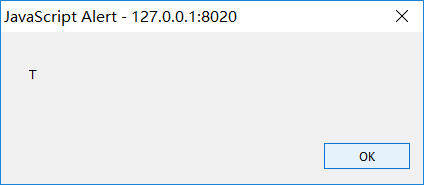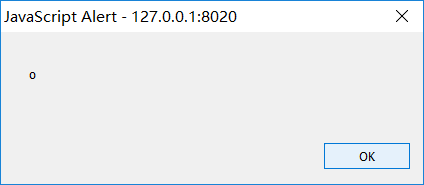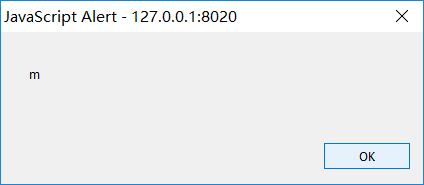上面这段JavaScript代码本以为要分别弹出Tom、John、Susan，但是浏览器运行后却弹出T、o、m。从理想状态中的字符串bug成了数组？

最后，当把‘name’改为name1后就完全没有问题了。

var name1 = ['Tom', 'John', 'Susan'];
for(var i = 0; i < 3; i++) {
}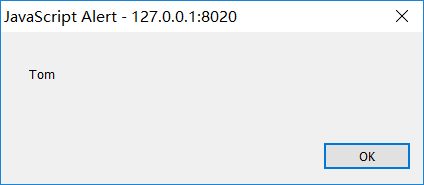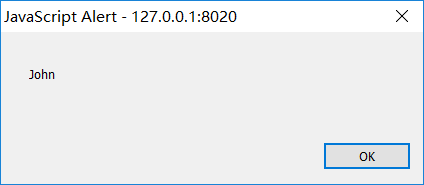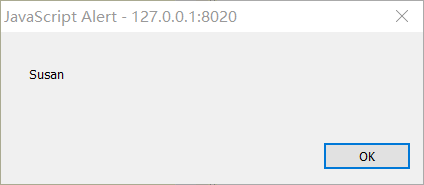经过测试和查找后，发现这一切都是name的问题,即命名的问题，其实name已经被JavaScript使用过了。

所以顺便又查了JavaScript的保留关键字，供方便即使避免：

JavaScript 保留关键字

在 JavaScript 中，一些标识符是保留关键字，不能用作变量名或函数名。

JavaScript 标准

所有的现代浏览器完全支持 ECMAScript 3（ES3，JavaScript 的第三版，从 1999 年开始）。

ECMAScript 4（ES4）未通过。

ECMAScript 5（ES5，2009 年发布），是 JavaScript 最新的官方版本。

随着时间的推移，我们开始看到，所有的现代浏览器已经完全支持 ES5。

JavaScript 保留关键字

Javascript 的保留关键字不可以用作变量、标签或者函数名。有些保留关键字是作为 Javascript 以后扩展使用。

abstract
arguments
boolean
break
byte
case
catch
char
class*
const
continue
debugger
default
delete
do
double
else
enum*
eval
export*
extends*
false
final
finally
float
for
function
goto
if
implements
import*
in
instanceof
int
interface
let
long
native
new
null
package
private
protected
public
return
short
static
super*
switch
synchronized
this
throw
throws
transient
true
try
typeof
var
void
volatile
while
with
yield

* 标记的关键字是 ECMAScript5 中新添加的。

JavaScript 对象、属性和方法

您也应该避免使用 JavaScript 内置的对象、属性和方法的名称作为 Javascript 的变量或函数名：

Array
Date
eval
function
hasOwnProperty
Infinity
isFinite
isNaN
isPrototypeOf
length
Math
NaN
name
Number
Object
prototype
String
toString
undefined
valueOf

Java 保留关键字

JavaScript 经常与 Java 一起使用。您应该避免使用一些 Java 对象和属性作为 JavaScript 标识符：

getClass
java
JavaArray
javaClass
JavaObject
JavaPackage

Windows 保留关键字

JavaScript 可以在 HTML 外部使用。它可在许多其他应用程序中作为编程语言使用。

在 HTML 中，您必须（为了可移植性，您也应该这么做）避免使用 HTML 和 Windows 对象和属性的名称作为 Javascript 的变量及函数名：

all
anchor
anchors
area
assign
blur
button
checkbox
clearInterval
clearTimeout
clientInformation
close
closed
confirm
constructor
crypto
decodeURI
decodeURIComponent
defaultStatus
document
element
elements
embed
embeds
encodeURI
encodeURIComponent
escape
event
focus
form
forms
frame
innerHeight
innerWidth
layer
layers
location
mimeTypes
navigate
navigator
frames
frameRate
hidden
history
image
images
offscreenBuffering
open
opener
option
outerHeight
outerWidth
packages
pageXOffset
pageYOffset
parent
parseFloat
parseInt
pkcs11
plugin
prompt
propertyIsEnum
reset
screenX
screenY
scroll
secure
select
self
setInterval
setTimeout
status
submit
taint
text
textarea
top
unescape
untaint
window

HTML 事件句柄

除此之外，您还应该避免使用 HTML 事件句柄的名称作为 Javascript 的变量及函数名。

实例：

onblur
onclick
onerror
onfocus
onkeydown
onkeypress
onkeyup
onmouseover
onmouseup
onmousedown
onsubmit

非标准 JavaScript

除了保留关键字，在 JavaScript 实现中也有一些非标准的关键字。

一个实例是 const 关键字，用于定义变量。 一些 JavaScript 引擎把 const 当作 var 的同义词。另一些引擎则把 const 当作只读变量的定义。

Const 是 JavaScript 的扩展。JavaScript 引擎支持它用在 Firefox 和 Chrome 中。但是它并不是 JavaScript 标准 ES3 或 ES5 的组成部分。建议：不要使用它。

展开全文name CSS 前端
• 字符串算法大整理！你想到的都找到（吧）。 2018.7.16 Chengdu 今天学习了字符串相关的一些算法，种类挺多的，特来整理一波。 字符串哈希(Hash) 简介 原理 哈希查找 字符串哈希 哈希的弊端 &amp...

字符串算法大整理！你能想到的都能找到（吧）。

2018.7.16 Chengdu

今天学习了字符串相关的一些算法，种类挺多的，特来整理一波。

字符串哈希(Hash)

简介
原理

哈希查找
字符串哈希

哈希的弊端 & 如何卡哈希

KMP算法

简介
1. 引言
2. 暴力匹配算法
3. KMP算法

3.1 定义
3.2 步骤
3.3 解释
3.3.1 寻找最长前缀后缀
3.3.2 基于《最大长度表》匹配
3.3.3 根据《最大长度表》求next 数组
3.3.4 通过代码递推计算next 数组
3.3.5 基于《next 数组》匹配
3.3.6 基于《最大长度表》与基于《next 数组》等价
3.3.7 Next 数组与有限状态自动机
3.3.8 Next 数组的优化

3.4 KMP的时间复杂度分析

4. 扩展1：BM算法
5. 扩展2：Sunday算法
6. 参考文献
7.后记
8.重编者（也就是我）记

字符串哈希(Hash)

简介

哈希( Hash$Hash$$Hash$ )是一种神奇的查找算法，广泛运用于计算机领域，它的强大在于设计得好的哈希算法可以使对一个对象的查找时间复杂度降为 O(1)$O\left(1\right)$$O(1)$ ，这是朴素查找的 O(n)$O\left(n\right)$$O(n)$ 和二分查找的 O(logn)$O\left(\mathrm{log}n\right)$$O(\log n)$ 所远不能及的。

第一次了解到哈希这一种技术是在吴军 dalao$dalao$$dalao$ 的《数学之美》一书上（感谢 MasterYin$MasterYin$$MasterYin$ 的借阅！）讲解网络爬虫的数学原理的一章。有兴趣的话可以去读一读。

原理

哈希查找

为什么哈希查找的时间复杂度可以这么低呢？考虑下面一个问题：

设计一个程序，以完成以下输入输出：
第一行，输入两个数字 n,m$n,m$$n,m$ 。
第二行，输入 n$n$$n$ 个数字。
第三行，输入 m$m$$m$ 个数字。对于每一个数字，询问其是否存在于第二行输入 n$n$$n$ 个数中，若是则输出”YES”，若不是则输出”NO”。
数据说明：输入的数字的范围在 [1,105]$\left[1,{10}^{5}\right]$$[1,10^5]$ 。

对于朴素算法，我们把第二行输入的数字储存在一个数组中。对于每一次询问，我们从左到右遍历整个数组来查询，每次询问的时间复杂度为 O(n)$O\left(n\right)$$O(n)$ 。

对于二分查找算法，我们把第二行输入的数字进行排序。对于每一次询问，我们用二分查找的方法找到这个数字处在的位置，排序的时间复杂度为 O(logn)$O\left(\mathrm{log}n\right)$$O(\log n)$ ，每次询问的时间复杂度也为 O(logn)$O\left(\mathrm{log}n\right)$$O(\log n)$ 。

可是这一题真的有这么复杂吗？我们可以使用一个大小为 105${10}^{5}$$10^5$ 的辅助数组 vis$vis$$vis$ 来表示一个数字是否出现过。对于第二行中输入的每一个数字 a$a$$a$ ，我们在 vis$vis$$vis$ 数组中进行记录： vis(a)=true$vis\left(a\right)=true$$vis(a)=true$ 。这样，对于每一次询问，我们只用 O(1)$O\left(1\right)$$O(1)$ 的时间访问 vis$vis$$vis$ 数组，就可以得到询问的结果了。而哈希，就是运用了这样的思想：我们多开一个数组，用这些多申请的空间去解决时间上的复杂。这也正是应了那句名言：

以空间换时间。 —-蒋介石

字符串哈希

那么如果我们把题改一下呢？

设计一个程序，以完成以下输入输出：
第一行，输入两个数字 n,m$n,m$$n,m$ 。
第二行，输入 n$n$$n$ 个字符串。
第三行，输入 m$m$$m$ 个字符串。对于每一个字符串，询问其是否存在于第二行输入 n$n$$n$ 个字符串中，若是则输出”YES”，若不是则输出”NO”。
数据说明：输入的字符串的长度范围在 [1,105]$\left[1,{10}^{5}\right]$$[1,10^5]$ 。

如果输入的是字符串的话，我们不就不能用 vis$vis$$vis$ 数组来储存了吗？这可怎么办？当然，如果你很熟悉 STL$STL$$STL$ 这一神奇的模板库，那么你一定会毫不犹豫地打出一行这样的代码：

map<string,bool>vis;

是的， map$map$$map$ 可以完美的解决这个问题。不过，我们可以试试用自己的力量来完成对字符串的处理：将字符串转换为数字。

怎样转换呢？我们知道，每一个字符都有它对应的 ASCII$ASCII$$ASCII$ 码，也就是说，一个字符可以表示为一个数字。那么，如果把字符串看作是字符的连续，不就可以把每个字符的 ASCII$ASCII$$ASCII$ 值连续起来表示字符串吗？我们可以运用这一点特性，将输入的字符串转换为一个 P$P$$P$ 进制数。在这里， P$P$$P$ 是一个大数。处理完后得到的 P$P$$P$ 进制数，就叫做这个字符串的哈希值。按照我的习惯，我会取 P=131$P=131$$P=131$ 。当然，如果你乐意，取点什么 P=233$P=233$$P=233$ 或 P=666$P=666$$P=666$ 也是可以的，不过很显然，计算难度也会随着 P$P$$P$ 的增大而逐步增大。

接下来给出一个函数 Hash$Hash$$Hash$ ，来获取一个字符串的哈希值：

const int P=131;

int Hash(string tmp)
{
int hash=0;
for(int i=0;i<tmp.length();i++)
hash=hash*P+tmp[i];
return hash;
}

可是我们又面临了一个问题，这是因为题目中写到：

数据说明：输入的字符串的长度范围在 [1,105]$\left[1,{10}^{5}\right]$$[1,10^5]$ 。

字符串的最大长度是 105${10}^{5}$$10^5$ ！那 int$int$$int$ 不就爆炸了吗？

所以我们还要定义一个大数 M$M$$M$ ，在 Hash$Hash$$Hash$ 函数的运算过程中令运算结果对它取模，作为最后的字符串哈希值。依照我的习惯，我会取 M=99991$M=99991$$M=99991$ 。当然，如果你乐意，取其它的值也是可以的。那么这个函数的运算就变成了：

const int P=131;
const int M=99991;

int Hash(string tmp)
{
int hash=0;
for(int i=0;i<tmp.length();i++)
hash=(hash*P+tmp[i])%M;
return hash;
}

这样我们就得到了一个字符串的哈希值，不过问题又出现了：怎样保证两个字符串的哈希各不相同？

这确实是哈希的一个大问题，具体来说有三种解决方法：

通过增加哈希池大小来降低两个字符串的哈希值冲突的概率。哈希池就是储存字符哈希值的地方，对应上述问题中的 vis$vis$$vis$ 数组。我们可以通过多模哈希或者增大 M$M$$M$ 的方式来达到这一结果，不过这样就会使得哈希计算过于复杂而且难以储存。
对于每个字符串的哈希值记录原有字符串，在冲突的情况下对原有字符串进行逐次比较。

第二种方法是我的常用方法，不过怎么实现呢？这里我们可以不使用 vis$vis$$vis$ 数组，而是转而使用神奇的 vector$vector$$vector$ 来达到这一点。不过这样的话，每次查找要调用一个函数 Add$Add$$Add$ ，每次询问要调用一个函数 Query$Query$$Query$ ，具体来说这样写：

vector<string>v[M];

bool Query(string tmp)
{
int pos=Hash(tmp);
for(int i=0;i<v[pos].size();i++)
if(v[pos][i]==tmp) return true;
return false;
}
{
if(Query(tmp)) return ;
int pos=Hash(tmp);
G[pos].push_back(tmp);
}

这样就达到了我们的目的。这里给出一道字符串哈希的模板题：

Luogu P3370 【模板】字符串哈希

哈希的弊端 & 如何卡哈希

可是还有的人为了图代码书写方便而拒绝使用强大的 vector$vector$$vector$ 来进行储存，这样就会被毒瘤出题人卡，因为会有人思考出卡掉各种字符串哈希的方法，具体可见几道神题：

BZOJ 3097 Hash Killer I
BZOJ 3098 Hash Killer II
BZOJ 3099 Hash Killer III
BZOJ 4917 [Lydsy1706月赛]Hash Killer IV (付费警告!)

在这些题目里，你被要扮演一个毒瘤出题人。因为你的后缀自动机神题被人用字符串哈希水掉了，所以你很不开心，决定用一组自造数据来卡掉他的代码。在这些题目中，给出水题的人的C++代码，要求你输出一组 hack$hack$$hack$ 数据。

题目挺有意思，但是我们如何来操作呢？即，我们如何构造出两个相同的字符串，使它们的哈希值相同呢？

先拿第一题做例子。下面是题目大意：

Hash killer 1：
本来的神题：给你一个长度为 N$N$$N$ 的字符串S$S$$S$ ，求有多少个不同的长度为 L$L$$L$ 的子串？
水过的人：给出一份 cpp ，其采用进制哈希配合 unsigned long long 的自然溢出来求出每一个长度为 L$L$$L$ 的子串的哈希值，然后使用排序去重得到不同的子串个数。
你的输出：你现在需要给出一组可以卡掉这个算法的数据。
数据大小：1≤N≤105$1\le N\le {10}^{5}$$1 \leq N \leq 10^5$ 。

自然溢出是什么意思呢？就是不去取 M$M$$M$ 的模，而是任由运算结果爆出数据范围，让储存范围自动地帮你取模。这也就相当于令 M=264$M={2}^{64}$$M=2^{64}$ 。他的代码如下：

typedef unsigned long long u64;
const int MaxN = 100000;
inline int hash_handle(const char *s, const int &n, const int &l, const int &base)
{
u64 hash_pow_l = 1;
for(int i=1;i<=l;i++)
hash_pow_l *= base;
int li_n = 0;
static u64 li[MaxN];
u64 val = 0;
for(int i=0;i<l;i++)
val=val*base+s[i]-'a';
li[li_n++]=val;
for(int i=l;i<n;i++)
{
val=val*base+s[i]-'a';
val-=(s[i-l]-'a')*hash_pow_l;
li[li_n++]=val;
}
sort(li,li+li_n);
li_n=unique(li,li+li_n)-li;
return li_n;
}

需要注意的事，这一代码中的基数 base$base$$base$ 值（也就是我代码中的 P$P$$P$ 值）是随机的。那么我们就需要分类讨论：

如果基数 base$base$$base$ 是偶数，那么 base$base$$base$ 就可以写作一个整数与 2$2$$2$ 相乘，而我们知道 M=264$M={2}^{64}$$M=2^{64}$ 。那么字符串长度超过 64$64$$64$ 即可卡掉。例如这两个字符串的哈希值相同：
"aaaaaaaaaaaaaaaaaaaaaaaaaaaaaaaaaaaaaaaaaaaaaaaaaaaaaaaaaaaaaaaaa"
"baaaaaaaaaaaaaaaaaaaaaaaaaaaaaaaaaaaaaaaaaaaaaaaaaaaaaaaaaaaaaaaa"
（别数了，两个字符串长度都是 65$65$$65$ ）
如果基数 base$base$$base$ 是奇数:：
我们可以构造两个字符串 str1 和 str2 ，我们队最终目的就是使它们满足 hash(str1)mod264=hash(str2)mod264$hash\left(str1\right)\phantom{\rule{0.667em}{0ex}}\mathrm{mod}\phantom{\rule{thinmathspace}{0ex}}\phantom{\rule{thinmathspace}{0ex}}{2}^{64}=hash\left(str2\right)\phantom{\rule{0.667em}{0ex}}\mathrm{mod}\phantom{\rule{thinmathspace}{0ex}}\phantom{\rule{thinmathspace}{0ex}}{2}^{64}$$hash(str1) \mod 2^{64} = hash(str2) \mod 2^{64}$ 。
即 (hash(str1)–hash(str2))mod264=0$\left(hash\left(str1\right)–hash\left(str2\right)\right)\phantom{\rule{0.667em}{0ex}}\mathrm{mod}\phantom{\rule{thinmathspace}{0ex}}\phantom{\rule{thinmathspace}{0ex}}{2}^{64}=0$$(hash(str1)–hash(str2)) \mod 2^{64}=0$ ，就能把代码卡掉。
令 01$01$$01$ 字符串 S[i]=S[i−1]+not(S[i−1]),S=′0′$S\left[i\right]=S\left[i-1\right]+not\left(S\left[i-1\right]\right),S\left[0\right]{=}^{\prime }{0}^{\prime }$$S[i]=S[i-1]+not(S[i-1]),S='0'$ 。其中，中括号中的 i$i$$i$ 仅表示字符串长度为 2i${2}^{i}$$2^i$ ， not(S[i−1])$not\left(S\left[i-1\right]\right)$$not(S[i-1])$ 表示对 01$01$$01$ 字符串 S[i−1]$S\left[i-1\right]$$S[i-1]$ 的每一位取反。
接下来定义 h(x)=hash(S[x]),hn(x)=hash(not(S[x]))$h\left(x\right)=hash\left(S\left[x\right]\right),hn\left(x\right)=hash\left(not\left(S\left[x\right]\right)\right)$$h(x)=hash(S[x]),hn(x)=hash(not(S[x]))$ 。
根据之前的定义以及进制哈希的特性，显然有：
h(i)–hn(i)=h(i−1)×base2i−1+hn(i−1)−hn(i−1)×base2i−1–h(i−1)=(h(i−1)–hn(i−1))×(base2i−1−1)$\begin{array}{rl}h\left(i\right)–hn\left(i\right)& =h\left(i-1\right)×bas{e}^{{2}^{i-1}}+hn\left(i-1\right)-hn\left(i-1\right)×bas{e}^{{2}^{i-1}}–h\left(i-1\right)\\ & =\left(h\left(i-1\right)–hn\left(i-1\right)\right)×\left(bas{e}^{{2}^{i-1}}-1\right)\end{array}$\begin{split}
h(i)–hn(i) & =h(i-1) \times base^{2^{i-1}}+hn(i-1)-hn(i-1) \times base^{2^{i-1}}–h(i-1)\\
& =(h(i-1)–hn(i-1)) \times (base^{2^{i-1}}-1)
\end{split}
对于 base2i−1−1$bas{e}^{{2}^{i-1}}-1$$base^{2^{i-1}}-1$ ，我们可以用神奇的欧拉定理得到：
==(base2i−1−1)mod2i(base0−1)mod2i0$\begin{array}{rl}& \left(bas{e}^{{2}^{i-1}}-1\right)\phantom{\rule{1em}{0ex}}\mathrm{mod}\phantom{\rule{thinmathspace}{0ex}}\phantom{\rule{thinmathspace}{0ex}}{2}^{i}\\ =& \left(bas{e}^{0}-1\right)\phantom{\rule{1em}{0ex}}\mathrm{mod}\phantom{\rule{thinmathspace}{0ex}}\phantom{\rule{thinmathspace}{0ex}}{2}^{i}\\ =& 0\end{array}$\begin{split}
& (base^{2^{i-1}}-1) \mod 2^i \\
= & (base^0-1) \mod 2^i \\
= & 0
\end{split}
所以我们就可以把上面的式子进行转换：
(h(i−1)–hn(i−1))×(base2i−1−1)=(h(i−1)–hn(i−1))×2ik$\left(h\left(i-1\right)–hn\left(i-1\right)\right)×\left(bas{e}^{{2}^{i-1}}-1\right)=\left(h\left(i-1\right)–hn\left(i-1\right)\right)×{2}^{i}k$(h(i-1)–hn(i-1)) \times (base^{2^{i-1}}-1)=(h(i-1)–hn(i-1)) \times 2^{i}k
其中的k是个没什么用的常数。然后我们用相同的方法拆啊拆啊拆，便可以得到：
==h(i)–hn(i)21+2+3+⋯+ik2(1+i)i2k$\begin{array}{rl}& h\left(i\right)–hn\left(i\right)\\ =& {2}^{1+2+3+\cdots +i}k\\ =& {2}^{\frac{\left(1+i\right)i}{2}}k\end{array}$\begin{split}
& h(i)–hn(i)\\
= & 2^{1+2+3+ \dots +i}k\\
= & 2^{\frac{(1+i)i}{2}}k
\end{split}
我们接下来只需要让 2(1+i)i2≥264${2}^{\frac{\left(1+i\right)i}{2}}\ge {2}^{6}4$$2^{\frac{(1+i)i}{2}} \geq 2^64$ 就好啦。经过简单的计算，我们要让 i≥11$i\ge 11$$i \geq 11$ 。

恭喜你，经过了层层计算，终于卡掉了他的代码！（体会到毒瘤出题人的艰辛）

接下来我们再来看看相对与计算无关的第二题吧。神题还是一模一样，只不过水题的人不打算使用自然溢出了，而是打算用模大质数的方法（这是剽窃我的代码啊喂）。而且喜欢大数字的他打算加大哈希池，令 M=109+7$M={10}^{9}+7$$M=10^9+7$ 。他的代码是这样的：

typedef unsigned long long u64;
const int MaxN = 100000;

inline int hash_handle(const char *s, const int &n, const int &l, const int &base)
{
const int Mod=1000000007;
u64 hash_pow_l=1;
for(int i=1;i<=l;i++)
hash_pow_l=(hash_pow_l*base)%Mod;
int li_n=0;
static int li[MaxN];
u64 val=0;
for(int i=0;i<l;i++)
val=(val*base+s[i]-'a')%Mod;
li[li_n++]=val;
for(int i=l;i<n;i++)
{
val=(val*base+s[i]-'a')%Mod;
val=(val+Mod-((s[i-l]-'a')*hash_pow_l)%Mod)%Mod;
li[li_n++]=val;
}
sort(li,li+li_n);
li_n =unique(li,li+li_n)-li;
return li_n;
}

这道题的做法也不难，但是要考验你的欧气了，先来了解一个神奇的悖论：

生日悖论_百度百科

那么同样的，如果你用大量的数据去卡他，他不就废了吗？不过如果你足够非，你还是可以WA到飞起的。

KMP算法

简介

三名算法大佬克努特（ D.E.Knuth$D.E.Knuth$$D.E.Knuth$ ）、莫里斯（ J.H.Morris$J.H.Morris$$J.H.Morris$ ）以及普拉特（ V.R.Pratt$V.R.Pratt$$V.R.Pratt$ ）改进了字符串匹配算法，所以我们合称这个算法为 KMP$KMP$$KMP$ 算法。网上对于这种算法有很多的讲解，而讲的最好的非这一篇莫属了。感谢这位大佬详尽的讲解。摘录于此，方便大家学习：

原文地址：v_JULY_v 的博客

1. 引言

本 KMP$KMP$$KMP$ 原文最初写于 2$2$$2$ 年多前的 2011$2011$$2011$ 年 12$12$$12$ 月，因当时初次接触 KMP$KMP$$KMP$ ，思路混乱导致写也写得混乱。所以一直想找机会重新写下 KMP$KMP$$KMP$ ，但苦于一直以来对 KMP$KMP$$KMP$ 的理解始终不够，故才迟迟没有修改本文。

然近期因开了个算法班，班上专门讲解数据结构、面试、算法，才再次仔细回顾了这个 KMP$KMP$$KMP$ ，在综合了一些网友的理解、以及算法班的两位讲师朋友曹博、邹博的理解之后，写了 9$9$$9$ 张 PPT$PPT$$PPT$ ，发在微博上。随后，一不做二不休，索性将 PPT$PPT$$PPT$ 上的内容整理到了本文之中（后来文章越写越完整，所含内容早已不再是九张 PPT$PPT$$PPT$ 那样简单了）。

KMP$KMP$$KMP$ 本身不复杂，但网上绝大部分的文章（包括本文的 2011$2011$$2011$ 年版本）把它讲混乱了。下面，咱们从暴力匹配算法讲起，随后阐述 KMP$KMP$$KMP$ 的流程、步骤、 next$next$$next$ 数组的简单求解、递推原理、代码求解，接着基于 next$next$$next$ 数组匹配，谈到有限状态自动机， next$next$$next$ 数组的优化， KMP$KMP$$KMP$ 的时间复杂度分析，最后简要介绍两个 KMP$KMP$$KMP$ 的扩展算法。

全文力图给你一个最为完整最为清晰的 KMP$KMP$$KMP$ ，希望更多的人不再被 KMP$KMP$$KMP$ 折磨或纠缠，不再被一些混乱的文章所混乱。有何疑问，欢迎随时留言评论， thanks$thanks$$thanks$ 。

2. 暴力匹配算法

假设现在我们面临这样一个问题：有一个文本串 S$S$$S$ ，和一个模式串 P$P$$P$ ，现在要查找 P$P$$P$ 在 S$S$$S$ 中的位置，怎么查找呢？

如果用暴力匹配的思路，并假设现在文本串 S$S$$S$ 匹配到 i$i$$i$ 位置，模式串 P$P$$P$ 匹配到 j$j$$j$ 位置，则有：
如果当前字符匹配成功（即 S[i] == P[j] ），则 i++,j++ ，继续匹配下一个字符；

如果失配（即 S[i] != P[j] ），令 i = i - (j - 1),j = 0 。相当于每次匹配失败时， i$i$$i$  回溯， j$j$$j$ 被置为 0$0$$0$ 。

理清楚了暴力匹配算法的流程及内在的逻辑，咱们可以写出暴力匹配的代码，如下：

int ViolentMatch(char* s, char* p)
{
int sLen = strlen(s);
int pLen = strlen(p);

int i = 0;
int j = 0;
while (i < sLen && j < pLen)
{
if (s[i] == p[j])
{
//如果当前字符匹配成功（即S[i] == P[j]），则i++，j++
i++;
j++;
}
else
{
//如果失配（即S[i]! = P[j]），令i = i - (j - 1)，j = 0
i = i - j + 1;
j = 0;
}
}
//匹配成功，返回模式串p在文本串s中的位置，否则返回-1
if (j == pLen)
return i - j;
else
return -1;
}

举个例子，如果给定文本串 S$S$$S$ "BBC ABCDAB ABCDABCDABDE" ，和模式串 P$P$$P$ "ABCDABD" ，现在要拿模式串 P$P$$P$ 去跟文本串 S$S$$S$ 匹配，整个过程如下所示：

S 为 B ， P 为 A ，不匹配，执行第②条指令：“如果失配（即 S[i] != P[j] ），令 i = i - (j - 1)，j = 0 ， S 跟 P 匹配，相当于模式串要往右移动一位（ i=1,j=0 ）。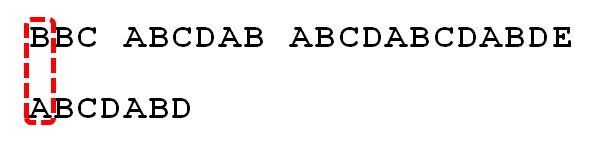S 跟 P 还是不匹配，继续执行第②条指令：如果失配（即 S[i] != P[j] ），令 i = i - (j - 1),j = 0 ， S 跟 P 匹配（ i=2,j=0 ），从而模式串不断的向右移动一位（不断的执行令 i = i - (j - 1),j = 0 ， i$i$$i$ 从 2$2$$2$ 变到 4$4$$4$ ， j$j$$j$ 一直为 0$0$$0$ ）。直到 S 跟 P 匹配成功（i=4,j=0），此时按照上面的暴力匹配算法的思路，转而执行第①条指令：如果当前字符匹配成功（即 S[i] == P[j] ），则 i++,j++ ，可得 S[i] 为 S ， P[j] 为 P ，即接下来 S 跟 P匹配（ i=5,j=1 ）。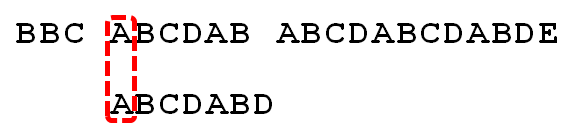S 跟 P 匹配成功，继续执行第①条指令：如果当前字符匹配成功（即 S[i] == P[j] ），则 i++,j++ ，得到 S 跟 P 匹配（ i=6,j=2 ），如此进行下去。直到 S 为空格字符， P 为字符 D （i=10,j=6 ），因为不匹配，重新执行第②条指令：“如果失配（即 S[i] != P[j] ），令 i = i - (j - 1),j = 0 ，相当于 S 跟 P 匹配（ i=5,j=0 ）。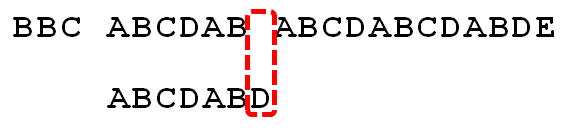至此，我们可以看到，如果按照暴力匹配算法的思路，尽管之前文本串和模式串已经分别匹配到了 S 、 P ，但因为 S 跟 P 不匹配，所以文本串回溯到 S ，模式串回溯到 P ，从而让 S 跟 P 匹配。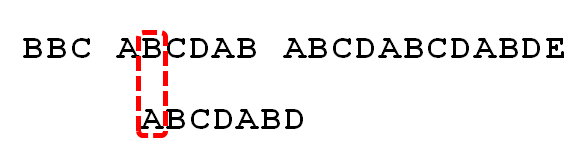而 S 肯定跟 P 失配。为什么呢？因为在之前第 4$4$$4$ 步匹配中，我们已经得知 S = P = B ，而 P = A ，即 P != P ，故 S 必定不等于 P ，所以回溯过去必然会导致失配。那有没有一种算法，让 i 不往回退，只需要移动 j 即可呢？

答案是肯定的。这种算法就是本文的主旨 KMP$KMP$$KMP$ 算法，它利用之前已经部分匹配这个有效信息，保持 i$i$$i$ 不回溯，通过修改 j$j$$j$ 的位置，让模式串尽量地移动到有效的位置。

3. KMP算法

3.1 定义

Knuth−Morris−Pratt$Knuth-Morris-Pratt$$Knuth-Morris-Pratt$ 字符串查找算法，简称为 “KMP$KMP$$KMP$算法”，常用于在一个文本串S$S$$S$内查找一个模式串P$P$$P$的出现位置，这个算法由DonaldKnuth$DonaldKnuth$$Donald Knuth$、VaughanPratt$VaughanPratt$$Vaughan Pratt$、JamesH.Morris$JamesH.Morris$$James H. Morris$三人于1977$1977$$1977$年联合发表，故取这3$3$$3$人的姓氏命名此算法。

下面先直接给出KMP$KMP$$KMP$的算法流程（如果感到一点点不适，没关系，坚持下，稍后会有具体步骤及解释，越往后看越会柳暗花明）：

假设现在文本串S$S$$S$匹配到i$i$$i$位置，模式串P$P$$P$匹配到j$j$$j$位置。

如果j = -1，或者当前字符匹配成功（即S[i] == P[j]），都令i++,j++，继续匹配下一个字符；
如果j != -1，且当前字符匹配失败（即S[i] != P[j]），则令i不变，j = next[j]。此举意味着失配时，模式串$P$相对于文本串$S$向右移动了j - next[j] 位。

换言之，当匹配失败时，模式串向右移动的位数为：失配字符所在位置 - 失配字符对应的next$next$$next$值（next$next$$next$数组的求解会在下文的3.3.3$3.3.3$$3.3.3$节中详细阐述），即移动的实际位数为：j−next[j]$j-next\left[j\right]$$j - next[j]$，且此值大于等于1$1$$1$。

很快，你也会意识到next$next$$next$数组各值的含义：代表当前字符之前的字符串中，有多大长度的相同前缀后缀。例如如果next[j]=k$next\left[j\right]=k$$next[j] = k$，代表j$j$$j$之前的字符串中有最大长度为k$k$$k$的相同前缀后缀。

此也意味着在某个字符失配时，该字符对应的next$next$$next$值会告诉你下一步匹配中，模式串应该跳到哪个位置（跳到next[j]$next\left[j\right]$$next[j]$的位置）。如果next [j] 等于0或-1，则跳到模式串的开头字符，若next[j]=k$next\left[j\right]=k$$next[j] = k$且k>0$k>0$$k > 0$，代表下次匹配跳到j$j$$j$之前的某个字符，而不是跳到开头，且具体跳过了k$k$$k$个字符。

转换成代码表示，则是：

int KmpSearch(char* s, char* p)
{
int i = 0;
int j = 0;
int sLen = strlen(s);
int pLen = strlen(p);
while (i < sLen && j < pLen)
{
//如果j = -1，或者当前字符匹配成功（即S[i] == P[j]），都令i++，j++
if (j == -1 || s[i] == p[j])
{
i++;
j++;
}
else
{
//如果j != -1，且当前字符匹配失败（即S[i] != P[j]），则令 i 不变，j = next[j]
//next[j]即为j所对应的next值
j = next[j];
}
}
if (j == pLen)
return i - j;
else
return -1;
}

继续拿之前的例子来说，当S跟P匹配失败时，KMP$KMP$$KMP$不是跟暴力匹配那样简单的把模式串右移一位，而是执行第②条指令：“如果j != -1，且当前字符匹配失败（即S[i] != P[j]），则令i不变，j = next[j]”，即j从6变到2（后面我们将求得P，即字符D对应的next值为2），所以相当于模式串向右移动的位数为j - next[j]（j - next[j] = 6-2 = 4）。向右移动4位后，S跟P继续匹配。为什么要向右移动4位呢，因为移动4位后，模式串中又有个“AB”可以继续跟S、S对应着，从而不用让i回溯。相当于在除去字符D的模式串子串中寻找相同的前缀和后缀，然后根据前缀后缀求出next数组，最后基于next数组进行匹配（不关心next数组是怎么求来的，只想看匹配过程是咋样的，可直接跳到下文3.3.4$3.3.4$$3.3.4$节）。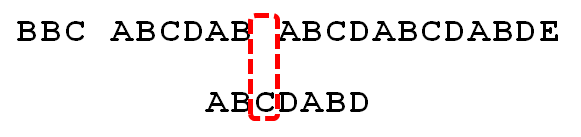3.2 步骤

①寻找前缀后缀最长公共元素长度

对于P=p[0,j]$P=p\left[0,j\right]$$P=p[0,j]$，寻找模式串P$P$$P$中长度最大且相等的前缀和后缀。如果存在p[0,k]=p[j−k,j]$p\left[0,k\right]=p\left[j-k,j\right]$$p[0,k]=p[j-k,j]$，那么在包含pj${p}_{j}$$p_j$的模式串中有最大长度为k+1$k+1$$k+1$的相同前缀后缀。举个例子，如果给定的模式串为“abab”，那么它的各个子串的前缀后缀的公共元素的最大长度如下表格所示：比如对于字符串"aba"来说，它有长度为1$1$$1$的相同前缀后缀a；而对于字符串abab来说，它有长度为2$2$$2$的相同前缀后缀ab（相同前缀后缀的长度为k+1$k+1$$k+1$，k+1=2$k+1=2$$k+1=2$）。

②求next$next$$next$数组

next$next$$next$数组考虑的是除当前字符外的最长相同前缀后缀，所以通过第①步骤求得各个前缀后缀的公共元素的最大长度后，只要稍作变形即可：将第①步骤中求得的值整体右移一位，然后初值赋为−1$-1$$-1$，如下表格所示：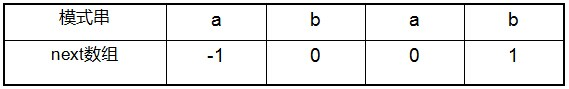比如对于aba来说，第3$3$$3$个字符a之前的字符串ab中有长度为0$0$$0$的相同前缀后缀，所以第3$3$$3$个字符a对应的next$next$$next$值为0$0$$0$；而对于abab来说，第4$4$$4$个字符b之前的字符串aba中有长度为1$1$$1$的相同前缀后缀a，所以第4$4$$4$个字符b对应的next$next$$next$值为1$1$$1$（相同前缀后缀的长度为k$k$$k$，k=1$k=1$$k=1$）。

③根据next$next$$next$数组进行匹配

匹配失配，j = next[j]，模式串向右移动的位数为：j - next[j]。换言之，当模式串的后缀p[j−k,j−1]$p\left[j-k,j-1\right]$$p[j-k,j-1]$跟文本串s[i−k,i−1]$s\left[i-k,i-1\right]$$s[i-k,i-1]$匹配成功，但pj${p}_{j}$$p_j$跟si${s}_{i}$$s_i$匹配失败时，因为next[j] = k，相当于在不包含pj${p}_{j}$$p_j$的模式串中有最大长度为k$k$$k$的相同前缀后缀，即p[0,k−1]=p[j−k,j−1]$p\left[0,k-1\right]=p\left[j-k,j-1\right]$$p[0,k-1] = p[j-k,j-1]$，故令j = next[j]，从而让模式串右移j - next[j]位，使得模式串的前缀p[0,k−1]$p\left[0,k-1\right]$$p[0,k-1]$对应着文本串s[i−k,i−1]$s\left[i-k,i-1\right]$$s[i-k,i-1]$，而后让pk${p}_{k}$$p_k$跟si${s}_{i}$$s_i$继续匹配。如下图所示：综上，KMP$KMP$$KMP$的next$next$$next$数组相当于告诉我们：当模式串中的某个字符跟文本串中的某个字符匹配失配时，模式串下一步应该跳到哪个位置。如模式串中在j$j$$j$处的字符跟文本串在i$i$$i$处的字符匹配失配时，下一步用next[j]$next\left[j\right]$$next[j]$处的字符继续跟文本串i$i$$i$处的字符匹配，相当于模式串向右移动j - next[j]位。

接下来，分别具体解释上述3$3$$3$个步骤。

3.3 解释

3.3.1 寻找最长前缀后缀

如果给定的模式串是：“ABCDABD”，从左至右遍历整个模式串，其各个子串的前缀后缀分别如下表格所示：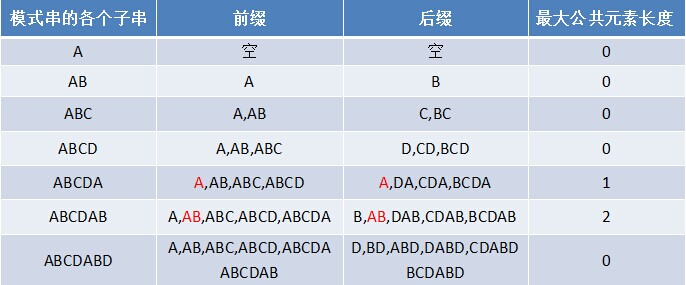也就是说，原模式串子串对应的各个前缀后缀的公共元素的最大长度表为（下简称《最大长度表》）：3.3.2 基于《最大长度表》匹配

因为模式串中首尾可能会有重复的字符，故可得出下述结论：

失配时，模式串向右移动的位数为：已匹配字符数 - 失配字符的上一位字符所对应的最大长度值。

下面，咱们就结合之前的《最大长度表》和上述结论，进行字符串的匹配。如果给定文本串“BBC ABCDAB ABCDABCDABDE”，和模式串“ABCDABD”，现在要拿模式串去跟文本串匹配，如下图所示：因为模式串中的字符A跟文本串中的字符B、B、C、空格一开始就不匹配，所以不必考虑结论，直接将模式串不断的右移一位即可，直到模式串中的字符A跟文本串的第5$5$$5$个字符A匹配成功：继续往后匹配，当模式串最后一个字符D跟文本串匹配时失配，显而易见，模式串需要向右移动。但向右移动多少位呢？因为此时已经匹配的字符数为6$6$$6$个（ABCDAB），然后根据《最大长度表》可得失配字符D的上一位字符B对应的长度值为2$2$$2$，所以根据之前的结论，可知需要向右移动6−2=4$6-2=4$$6-2=4$位。模式串向右移动4$4$$4$位后，发现C处再度失配，因为此时已经匹配了2$2$$2$个字符（AB），且上一位字符B对应的最大长度值为0$0$$0$，所以向右移动：2−0=2$2-0=2$$2-0=2$位。A与空格失配，向右移动1$1$$1$位。继续比较，发现D与C失配，故向右移动的位数为：已匹配的字符数6$6$$6$减去上一位字符B对应的最大长度2$2$$2$，即向右移动6−2=4$6-2=4$$6-2=4$位。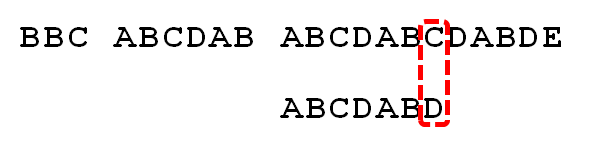经历第5$5$$5$步后，发现匹配成功，过程结束。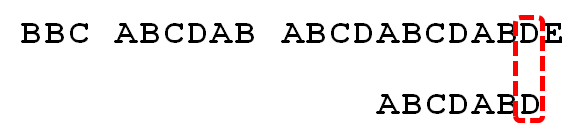通过上述匹配过程可以看出，问题的关键就是寻找模式串中最大长度的相同前缀和后缀，找到了模式串中每个字符之前的前缀和后缀公共部分的最大长度后，便可基于此匹配。而这个最大长度便正是next$next$$next$数组要表达的含义。

3.3.3 根据《最大长度表》求next 数组

由上文，我们已经知道，字符串“ABCDABD”各个前缀后缀的最大公共元素长度分别为：而且，根据这个表可以得出下述结论

失配时，模式串向右移动的位数为：已匹配字符数 - 失配字符的上一位字符所对应的最大长度值

上文利用这个表和结论进行匹配时，我们发现，当匹配到一个字符失配时，其实没必要考虑当前失配的字符，更何况我们每次失配时，都是看的失配字符的上一位字符对应的最大长度值。如此，便引出了next$next$$next$数组。

给定字符串“ABCDABD”，可求得它的next$next$$next$数组如下：把next$next$$next$数组跟之前求得的最大长度表对比后，不难发现，next$next$$next$数组相当于“最大长度值” 整体向右移动一位，然后初始值赋为−1$-1$$-1$。意识到了这一点，你会惊呼原来next 数组的求解竟然如此简单：就是找最大对称长度的前缀后缀，然后整体右移一位，初值赋为−1$-1$$-1$（当然，你也可以直接计算某个字符对应的next$next$$next$值，就是看这个字符之前的字符串中有多大长度的相同前缀后缀）。

换言之，对于给定的模式串：ABCDABD，它的最大长度表及next$next$$next$数组分别如下：根据最大长度表求出了next$next$$next$数组后，从而有

失配时，模式串向右移动的位数为：失配字符所在位置 - 失配字符对应的next$next$$next$值

而后，你会发现，无论是基于《最大长度表》的匹配，还是基于next$next$$next$数组的匹配，两者得出来的向右移动的位数是一样的。为什么呢？因为：

根据《最大长度表》，失配时，模式串向右移动的位数 = 已经匹配的字符数 - 失配字符的上一位字符的最大长度值。
而根据《next$next$$next$数组》，失配时，模式串向右移动的位数 = 失配字符的位置 - 失配字符对应的next$next$$next$值。

其中，从0$0$$0$开始计数时，失配字符的位置 = 已经匹配的字符数（失配字符不计数），而失配字符对应的next$next$$next$值 = 失配字符的上一位字符的最大长度值，两相比较，结果必然完全一致。

所以，你可以把《最大长度表》看做是next$next$$next$数组的雏形，甚至就把它当做next$next$$next$数组也是可以的，区别不过是怎么用的问题。

3.3.4 通过代码递推计算next 数组

接下来，咱们来写代码求下next$next$$next$数组。

基于之前的理解，可知计算next$next$$next$数组的方法可以采用递推：

1.如果对于值k$k$$k$，已有p[0,k−1]=p[j−k,j−1]$p\left[0,k-1\right]=p\left[j-k,j-1\right]$$p[0,k-1]=p[j-k,j-1]$，相当于next[j]=k$next\left[j\right]=k$$next[j]=k$。

此意味着什么呢？究其本质，next[j] = k代表p[j]之前的模式串子串中，有长度为k$k$$k$的相同前缀和后缀。有了这个next$next$$next$数组，在KMP$KMP$$KMP$匹配中，当模式串中j处的字符失配时，下一步用next[j]处的字符继续跟文本串匹配，相当于模式串向右移动j - next[j]位。

举个例子，如下图，根据模式串“ABCDABD”的next$next$$next$数组可知失配位置的字符D对应的next$next$$next$值为2$2$$2$，代表字符D前有长度为2$2$$2$的相同前缀和后缀（这个相同的前缀后缀即为“AB”），失配后，模式串需要向右移动j - next [j] = 6 - 2 =4位。向右移动4$4$$4$位后，模式串中的字符C继续跟文本串匹配。2.下面的问题是：已知next[0,j]$next\left[0,j\right]$$next[0,j]$，如何求出next[j+1]$next\left[j+1\right]$$next[j+1]$呢？

对于P$P$$P$的前j+1$j+1$$j+1$个序列字符：

若p[k] == p[j]，则next[j + 1] = next[j] + 1 = k + 1；
若p[k] != p[j]，如果此时p[next[k]] == p[j]，则next[ j + 1 ] =  next[k] + 1，否则继续递归前缀索引k = next[k]，而后重复此过程。 相当于在字符p[j+1]之前不存在长度为k+1$k+1$$k+1$的前缀p[0,k]$p\left[0,k\right]$$p[0,k]$跟后缀p[j−k,j]$p\left[j-k,j\right]$$p[j-k,j]$相等，那么是否可能存在另一个值t+1<k+1$t+1$t+1，使得长度更小的前缀p[0,t]$p\left[0,t\right]$$p[0,t]$等于长度更小的后缀p[j−t,j]$p\left[j-t,j\right]$$p[j-t,j]$呢？如果存在，那么这个t+1$t+1$$t+1$便是next[j+1]$next\left[j+1\right]$$next[j+1]$的值，此相当于利用已经求得的next$next$$next$数组（next[0,…,k,…,j]$next\left[0,\dots ,k,\dots ,j\right]$$next[0, \dots ,k, \dots ,j]$）进行P$P$$P$串前缀跟P$P$$P$串后缀的匹配。

一般的文章或教材可能就此一笔带过，但大部分的初学者可能还是不能很好的理解上述求解next$next$$next$数组的原理，故接下来，我再来着重说明下。

如下图所示，假定给定模式串ABCDABCE，且已知next[j]=k$next\left[j\right]=k$$next[j] = k$（相当于p[0,k−1]=p[j−k,j−1]=AB$p\left[0,k-1\right]=p\left[j-k,j-1\right]=AB$$p[0,k-1]=p[j-k,j-1]=AB$，可以看出k$k$$k$为2$2$$2$），现要求next[j+1]$next\left[j+1\right]$$next[j+1]$等于多少？因为pk=pj=′C′${p}_{k}={p}_{j}{=}^{\prime }{C}^{\prime }$$p_k=p_j='C'$，所以next[j+1]=next[j]+1=k+1$next\left[j+1\right]=next\left[j\right]+1=k+1$$next[j+1]=next[j]+1=k+1$（可以看出next[j+1]=3$next\left[j+1\right]=3$$next[j+1]=3$）。代表字符E前的模式串中，有长度k+1$k+1$$k+1$的相同前缀后缀。但如果p[k] != p[j]呢？说明p[0,k]≠p[j−k,j]$p\left[0,k\right]\ne p\left[j-k,j\right]$$p[0,k] \neq p[j-k,j]$。换言之，当p[k] != p[j]后，字符E前有多大长度的相同前缀后缀呢？很明显，因为C不同于D，所以ABC跟ABD不相同，即字符E前的模式串没有长度为k+1的相同前缀后缀，也就不能再简单地令：next[j + 1] = next[j] + 1。所以，咱们只能去寻找长度更短一点的相同前缀后缀。结合上图来讲，若能在前缀p[0,k]$p\left[0,k\right]$$p[0,k]$中不断的递归前缀索引k=next[k]$k=next\left[k\right]$$k = next[k]$，找到一个字符pk′${p}_{{k}^{\prime }}$$p_{k’}$也为D，代表pk′=pj${p}_{{k}^{\prime }}={p}_{j}$$p_{k’} = p_j$，且满足p[0,k′]=p[j−k′,j]$p\left[0,{k}^{\prime }\right]=p\left[j-{k}^{\prime },j\right]$$p[0,k'] = p[j-k',j]$，则最大相同的前缀后缀长度为k′+1${k}^{\prime }+1$$k' + 1$，从而next[j+1]=k′+1=next[k′]+1$next\left[j+1\right]={k}^{\prime }+1=next\left[{k}^{\prime }\right]+1$$next[j + 1] = k’ + 1 = next[k'] + 1$。否则前缀中没有D，则代表没有相同的前缀后缀，next[j+1]=0$next\left[j+1\right]=0$$next[j + 1] = 0$。

那为何递归前缀索引k=next[k]$k=next\left[k\right]$$k = next[k]$，就能找到长度更短的相同前缀后缀呢？这又归根到next$next$$next$数组的含义。我们拿前缀p[0,k]$p\left[0,k\right]$$p[0,k]$去跟后缀p[j−k,j]$p\left[j-k,j\right]$$p[j-k,j]$匹配，如果pk${p}_{k}$$p_k$跟pj${p}_{j}$$p_j$失配，下一步就是用p[next[k]]$p\left[next\left[k\right]\right]$$p[next[k]]$去跟pj${p}_{j}$$p_j$继续匹配，如果p[next[k]]$p\left[next\left[k\right]\right]$$p[next[k]]$跟pj${p}_{j}$$p_j$还是不匹配，则需要寻找长度更短的相同前缀后缀，即下一步用p[next[next[k]]]$p\left[next\left[next\left[k\right]\right]\right]$$p[next[next[k]]]$去跟pj${p}_{j}$$p_j$匹配。此过程相当于模式串的自我匹配，所以不断的递归k=next[k]$k=next\left[k\right]$$k=next[k]$，直到要么找到长度更短的相同前缀后缀，要么没有长度更短的相同前缀后缀。如下图所示：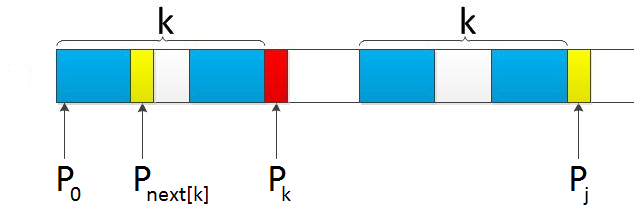所以，因最终在前缀ABC中没有找到D，故E的next$next$$next$值为0$0$$0$：

模式串的后缀：ABDE
模式串的前缀：ABC
前缀右移两位：     ABC

读到此，有的读者可能又有疑问了，那能否举一个能在前缀中找到字符D的例子呢？OK，咱们便来看一个能在前缀中找到字符D的例子，如下图所示：给定模式串DABCDABDE，我们很顺利的求得字符D之前的“DABCDAB”的各个子串的最长相同前缀后缀的长度分别为0 0 0 0 1 2 3，但当遍历到字符D，要求包括D在内的“DABCDABD”最长相同前缀后缀时，我们发现pj${p}_{j}$$p_j$处的字符D跟pk${p}_{k}$$p_k$处的字符C不一样，换言之，前缀DABC的最后一个字符C跟后缀DABD的最后一个字符D不相同，所以不存在长度为4$4$$4$的相同前缀后缀。

怎么办呢？既然没有长度为4$4$$4$的相同前缀后缀，咱们可以寻找长度短点的相同前缀后缀，最终，因在p0${p}_{0}$$p_0$处发现也有个字符D，p0=pj${p}_{0}={p}_{j}$$p_0 = p_j$，所以p[j]对应的长度值为1$1$$1$，相当于E对应的next$next$$next$值为1$1$$1$（即字符E之前的字符串“DABCDABD”中有长度为1$1$$1$的相同前缀和后缀）。

综上，可以通过递推求得next$next$$next$数组，代码如下所示：

void GetNext(char* p,int next[])
{
int pLen = strlen(p);
next = -1;
int k = -1;
int j = 0;
while (j < pLen - 1)
{
//p[k]表示前缀，p[j]表示后缀
if (k == -1 || p[j] == p[k])
{
++k;
++j;
next[j] = k;
}
else
{
k = next[k];
}
}
}

用代码重新计算下“ABCDABD”的next$next$$next$数组，以验证之前通过“最长相同前缀后缀长度值右移一位，然后初值赋为−1$-1$$-1$”得到的next$next$$next$数组是否正确，计算结果如下表格所示：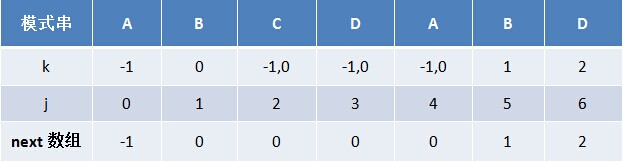从上述表格可以看出，无论是之前通过“最长相同前缀后缀长度值右移一位，然后初值赋为−1$-1$$-1$”得到的next$next$$next$数组，还是之后通过代码递推计算求得的next$next$$next$数组，结果是完全一致的。

3.3.5 基于《next 数组》匹配

下面，我们来基于next$next$$next$数组进行匹配。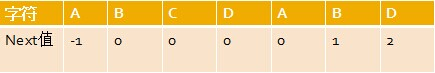还是给定文本串“BBC ABCDAB ABCDABCDABDE”，和模式串“ABCDABD”，现在要拿模式串去跟文本串匹配，如下图所示：在正式匹配之前，让我们来再次回顾下上文2.1$2.1$$2.1$节所述的KMP$KMP$$KMP$算法的匹配流程：

“假设现在文本串S$S$$S$匹配到i$i$$i$位置，模式串P$P$$P$匹配到j$j$$j$位置

如果j == -1，或者当前字符匹配成功（即S[i] == P[j]），都令i++,j++，继续匹配下一个字符；
如果j != -1，且当前字符匹配失败（即S[i] != P[j]），则令i不变，j = next[j]。此举意味着失配时，模式串P$P$$P$相对于文本串S$S$$S$向右移动了j - next[j]位。
换言之，当匹配失败时，模式串向右移动的位数为：失配字符所在位置 - 失配字符对应的next$next$$next$值，即移动的实际位数为：j - next[j]，且此值大于等于1$1$$1$。”
1.最开始匹配时

P$P\left[0\right]$$P$跟S$S\left[0\right]$$S$匹配失败
所以执行“如果j != -1，且当前字符匹配失败（即S[i] != P[j]），则令i不变，j = next[j]”，所以j = -1，故转而执行“如果j = -1，或者当前字符匹配成功（即S[i]==P[j]$S\left[i\right]==P\left[j\right]$$S[i] == P[j]$），都令i++,j++”，得到i = 1,j = 0，即P$P\left[0\right]$$P$继续跟S$S\left[1\right]$$S$匹配。
P$P\left[0\right]$$P$跟S$S\left[1\right]$$S$又失配，j$j$$j$再次等于−1$-1$$-1$，i$i$$i$、j$j$$j$继续自增，从而P$P\left[0\right]$$P$跟S$S\left[2\right]$$S$匹配。
P$P\left[0\right]$$P$跟S$S\left[2\right]$$S$失配后，P$P\left[0\right]$$P$又跟S$S\left[3\right]$$S$匹配。
P$P\left[0\right]$$P$跟S$S\left[3\right]$$S$再失配，直到P$P\left[0\right]$$P$跟S$S\left[4\right]$$S$匹配成功，开始执行此条指令的后半段：“如果j == -1，或者当前字符匹配成功（即S[i]==P[j]$S\left[i\right]==P\left[j\right]$$S[i] == P[j]$），都令i++,j++”。2.P$P\left[1\right]$$P$跟S$S\left[5\right]$$S$匹配成功，P$P\left[2\right]$$P$跟S$S\left[6\right]$$S$也匹配成功, …，直到当匹配到P$P\left[6\right]$$P$处的字符D时失配（即S!=P$S\left[10\right]!=P\left[6\right]$$S != P$），由于P$P\left[6\right]$$P$处的D对应的next$next$$next$值为2$2$$2$，所以下一步用P$P\left[2\right]$$P$处的字符C继续跟S$S\left[10\right]$$S$匹配，相当于向右移动：j−next[j]=6−2=4$j-next\left[j\right]=6-2=4$$j - next[j] = 6 - 2 =4$位。3.向右移动4$4$$4$位后，P$P\left[2\right]$$P$处的C再次失配，由于C对应的next$next$$next$值为0$0$$0$，所以下一步用P$P\left[0\right]$$P$处的字符继续跟S$S\left[10\right]$$S$匹配，相当于向右移动：j−next[j]=2−0=2$j-next\left[j\right]=2-0=2$$j - next[j] = 2 - 0 = 2$位。4.移动两位之后，A$A$$A$跟空格不匹配，模式串后移1$1$$1$位。5.P$P\left[6\right]$$P$处的D再次失配，因为P$P\left[6\right]$$P$对应的next$next$$next$值为2$2$$2$，故下一步用P$P\left[2\right]$$P$继续跟文本串匹配，相当于模式串向右移动j - next[j] = 6 - 2 = 4位。6.匹配成功，过程结束。匹配过程一模一样。也从侧面佐证了，next$next$$next$数组确实是只要将各个最大前缀后缀的公共元素的长度值右移一位，且把初值赋为−1$-1$$-1$即可。

3.3.6 基于《最大长度表》与基于《next 数组》等价

我们已经知道，利用next$next$$next$数组进行匹配失配时，模式串向右移动j - next[j]位，等价于已匹配字符数 - 失配字符的上一位字符所对应的最大长度值。原因是：

j$j$$j$从0$0$$0$开始计数，那么当数到失配字符时，j$j$$j$的数值就是已匹配的字符数；
由于next$next$$next$数组是由最大长度值表整体向右移动一位（且初值赋为−1$-1$$-1$）得到的，那么失配字符的上一位字符所对应的最大长度值，即为当前失配字符的next$next$$next$值。

但为何本文不直接利用next$next$$next$数组进行匹配呢？因为next$next$$next$数组不好求，而一个字符串的前缀后缀的公共元素的最大长度值很容易求。例如若给定模式串“ababa”，要你快速口算出其next$next$$next$数组，乍一看，每次求对应字符的next$next$$next$值时，还得把该字符排除之外，然后看该字符之前的字符串中有最大长度为多大的相同前缀后缀，此过程不够直接。而如果让你求其前缀后缀公共元素的最大长度，则很容易直接得出结果：0 0 1 2 3，如下表格所示：然后这5$5$$5$个数字 全部整体右移一位，且初值赋为−1$-1$$-1$，即得到其next$next$$next$数组：-1 0 0 1 2。

3.3.7 Next 数组与有限状态自动机

next$next$$next$负责把模式串向前移动，且当第j位不匹配的时候，用第next[j]$next\left[j\right]$$next[j]$位和主串匹配，就像打了张“表”。此外，next$next$$next$也可以看作有限状态自动机的状态，在已经读了多少字符的情况下，失配后，前面读的若干个字符是有用的。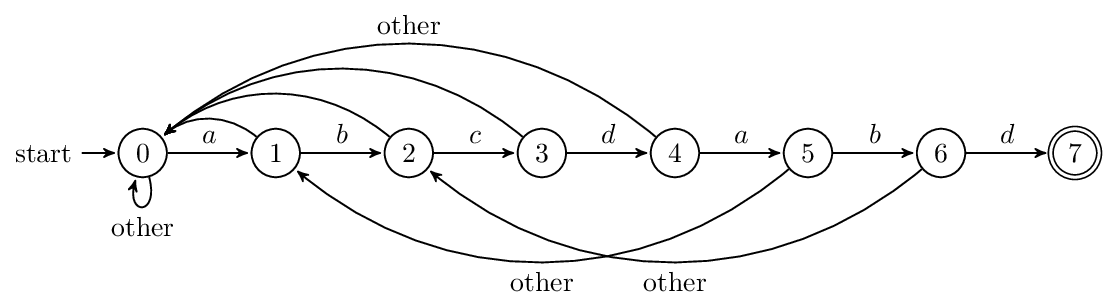3.3.8 Next 数组的优化

行文至此，咱们全面了解了暴力匹配的思路、KMP$KMP$$KMP$算法的原理、流程、流程之间的内在逻辑联系，以及next$next$$next$数组的简单求解（《最大长度表》整体右移一位，然后初值赋为−1$-1$$-1$）和代码求解，最后基于《next$next$$next$数组》的匹配，看似洋洋洒洒，清晰透彻，但以上忽略了一个小问题。

比如，如果用之前的next 数组方法求模式串“abab”的next 数组，可得其next 数组为-1 0 0 1（0 0 1 2整体右移一位，初值赋为−1$-1$$-1$），当它跟下图中的文本串去匹配的时候，发现b跟c失配，于是模式串右移j - next[j] = 3 - 1 = 2位。右移2$2$$2$位后，b又跟c失配。事实上，因为在上一步的匹配中，已经得知p=b$p\left[3\right]=b$$p=b$，与s=c$s\left[3\right]=c$$s=c$失配，而右移两位之后，让p[next]=p=b$p\left[next\left[3\right]\right]=p\left[1\right]=b$$p[next]=p=b$再跟s$s\left[3\right]$$s$匹配时，必然失配。问题出在哪呢？问题出在不该出现p[j]=p[next[j]]$p\left[j\right]=p\left[next\left[j\right]\right]$$p[j]=p[next[j]]$。为什么呢？理由是：当p[j]≠s[i]$p\left[j\right]\ne s\left[i\right]$$p[j] \neq s[i]$时，下次匹配必然是p[next[j]]$p\left[next\left[j\right]\right]$$p[next[j]]$跟s[i]$s\left[i\right]$$s[i]$匹配，如果p[j]=p[next[j]]$p\left[j\right]=p\left[next\left[j\right]\right]$$p[j]=p[next[j]]$，必然导致后一步匹配失败（因为p[j]$p\left[j\right]$$p[j]$已经跟s[i]$s\left[i\right]$$s[i]$失配，然后你还用跟p[j]$p\left[j\right]$$p[j]$等同的值p[next[j]]$p\left[next\left[j\right]\right]$$p[next[j]]$去跟s[i]$s\left[i\right]$$s[i]$匹配，很显然，必然失配），所以不能允许p[j]=p[next[j]]$p\left[j\right]=p\left[next\left[j\right]\right]$$p[j]=p[next[j]]$。如果出现了p[j]=p[next[j]]$p\left[j\right]=p\left[next\left[j\right]\right]$$p[j]=p[next[j]]$咋办呢？如果出现了，则需要再次递归，即令next[j]=next[next[j]]$next\left[j\right]=next\left[next\left[j\right]\right]$$next[j]=next[next[j]]$。

所以，咱们得修改下求next$next$$next$数组的代码。

//优化过后的next 数组求法
void GetNextval(char* p, int next[])
{
int pLen = strlen(p);
next = -1;
int k = -1;
int j = 0;
while (j < pLen - 1)
{
//p[k]表示前缀，p[j]表示后缀
if (k == -1 || p[j] == p[k])
{
++j;
++k;
//较之前next数组求法，改动在下面4行
if (p[j] != p[k])
next[j] = k;   //之前只有这一行
else
//因为不能出现p[j] = p[ next[j ]]，所以当出现时需要继续递归，k = next[k] = next[next[k]]
next[j] = next[k];
}
else
{
k = next[k];
}
}
}

利用优化过后的next$next$$next$数组求法，可知模式串“abab”的新next$next$$next$数组为：-1 0 -1 0。可能有些读者会问：原始next$next$$next$数组是前缀后缀最长公共元素长度值右移一位， 然后初值赋为−1$-1$$-1$而得，那么优化后的next$next$$next$数组如何快速心算出呢？实际上，只要求出了原始next$next$$next$数组，便可以根据原始next$next$$next$数组快速求出优化后的next$next$$next$数组。还是以abab为例，如下表格所示：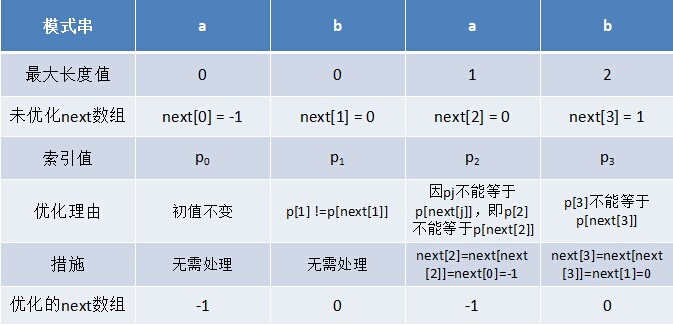只要出现了p[next[j]]=p[j]$p\left[next\left[j\right]\right]=p\left[j\right]$$p[next[j]]=p[j]$的情况，则把next[j]$next\left[j\right]$$next[j]$的值再次递归。例如在求模式串“abab”的第2$2$$2$个a的next$next$$next$值时，如果是未优化的next$next$$next$值的话，第2$2$$2$个a对应的next$next$$next$值为0$0$$0$，相当于第2$2$$2$个a失配时，下一步匹配模式串会用p$p\left[0\right]$$p$处的a再次跟文本串匹配，必然失配。所以求第2$2$$2$个a的next$next$$next$值时，需要再次递归：next = next[ next ] = next = -1（此后，根据优化后的新next$next$$next$值可知，第2$2$$2$个a失配时，执行“如果j == -1，或者当前字符匹配成功（即S[i] == P[j]），都令i++,j++，继续匹配下一个字符”），同理，第2$2$$2$个b对应的next$next$$next$值为0$0$$0$。

对于优化后的next$next$$next$数组可以发现一点：如果模式串的后缀跟前缀相同，那么它们的next$next$$next$值也是相同的，例如模式串abcabc，它的前缀后缀都是abc，其优化后的next$next$$next$数组为：-1 0 0 -1 0 0，前缀后缀abc的next$next$$next$值都为-1 0 0。

然后引用下之前3.1$3.1$$3.1$节的KMP$KMP$$KMP$代码：

int KmpSearch(char* s, char* p)
{
int i = 0;
int j = 0;
int sLen = strlen(s);
int pLen = strlen(p);
while (i < sLen && j < pLen)
{
//①如果j = -1，或者当前字符匹配成功（即S[i] == P[j]），都令i++，j++
if (j == -1 || s[i] == p[j])
{
i++;
j++;
}
else
{
//②如果j != -1，且当前字符匹配失败（即S[i] != P[j]），则令 i 不变，j = next[j]
//next[j]即为j所对应的next值
j = next[j];
}
}
if (j == pLen)
return i - j;
else
return -1;
}

接下来，咱们继续拿之前的例子说明，整个匹配过程如下：

S$S\left[3\right]$$S$与P$P\left[3\right]$$P$匹配失败。S$S\left[3\right]$$S$保持不变，P$P$$P$的下一个匹配位置是P[next]$P\left[next\left[3\right]\right]$$P[next]$，而next=0$next\left[3\right]=0$$next=0$，所以P[next]=P$P\left[next\left[3\right]\right]=P\left[0\right]$$P[next]=P$与S$S\left[3\right]$$S$匹配。由于上一步骤中P$P\left[0\right]$$P$与S$S\left[3\right]$$S$还是不匹配。此时i=3$i=3$$i=3$，j=next=−1$j=next\left[0\right]=-1$$j=next =-1$，由于满足条件j==−1$j==-1$$j==-1$，所以执行“++i,++j”，即主串指针下移一个位置，P$P\left[0\right]$$P$与S$S\left[4\right]$$S$开始匹配。最后j==pLen，跳出循环，输出结果i - j = 4（即模式串第一次在文本串中出现的位置），匹配成功，算法结束。3.4 KMP的时间复杂度分析

相信大部分读者读完上文之后，已经发觉其实理解$KMP$非常容易，无非是循序渐进把握好下面几点：

如果模式串中存在相同前缀和后缀，即$p[j-k,j-1]=p[0,k-1]$，那么在$p_j$跟$s_i$失配后，让模式串的前缀$p[0,k-1]$对应着文本串$s[i-k,i-1]$，而后让$p_k$跟$s_i$继续匹配。
之前本应是$p_j$跟$s_i$匹配，结果失配了，失配后，令$p_k$跟$s_i$匹配，相当于$j$变成了$k$，模式串向右移动$j-k$位。
因为k 的值是可变的，所以我们用$next[j]$表示$j$处字符失配后，下一次匹配模式串应该跳到的位置。换言之，失配前是$j$，$p_j$跟$s_i$失配时，用$p[next[j]]$继续跟$s_i$匹配，相当于$j$变成了$next[j]$，所以，$j = next[j]$，等价于把模式串向右移动$j - next[j]$位。
而$next[j]$应该等于多少呢？$next[j]$的值由$j$之前的模式串子串中有多大长度的相同前缀后缀所决定，如果$j$之前的模式串子串中（不含$j$）有最大长度为$k$的相同前缀后缀，那么$next[j] = k$。

如之前的图所示：接下来，咱们来分析下$KMP$的时间复杂度。分析之前，先来回顾下$KMP$匹配算法的流程：

$KMP$的算法流程：
假设现在文本串$S$匹配到$i$位置，模式串$P$匹配到$j$位置
如果j == -1，或者当前字符匹配成功（即S[i] == P[j]），都令i++,j++，继续匹配下一个字符；
如果j != -1，且当前字符匹配失败（即S[i] != P[j]），则令i不变，j = next[j]。此举意味着失配时，模式串$P$相对于文本串$S$向右移动了j - next [j]位。

我们发现如果某个字符匹配成功，模式串首字符的位置保持不动，仅仅是i++,j++；如果匹配失配，i不变（即i不回溯），模式串会跳过匹配过的next[j]个字符。整个算法最坏的情况是，当模式串首字符位于i - j的位置时才匹配成功，算法结束。

所以，如果文本串的长度为$n$，模式串的长度为$m$，那么匹配过程的时间复杂度为$O(n)$，算上计算$next$的$O(m)$时间，$KMP$的整体时间复杂度为$O(m+n)$。

4. 扩展1：BM算法

KMP$KMP$$KMP$ 的匹配是从模式串的开头开始匹配的，而 1977$1977$$1977$ 年，德克萨斯大学的 RobertS.Boyer$RobertS.Boyer$$Robert S. Boyer$ 教授和 JStrotherMoore$JStrotherMoore$$J Strother Moore$ 教授发明了一种新的字符串匹配算法： Boyer−Moore$Boyer-Moore$$Boyer-Moore$ 算法，简称BM$BM$$BM$ 算法。该算法从模式串的尾部开始匹配，且拥有在最坏情况下 O(N)$O\left(N\right)$$O(N)$ 的时间复杂度。在实践中，比 KMP$KMP$$KMP$ 算法的实际效能高。

BM$BM$$BM$ 算法定义了两个规则：

坏字符规则：当文本串中的某个字符跟模式串的某个字符不匹配时，我们称文本串中的这个失配字符为坏字符，此时模式串需要向右移动，移动的位数 = 坏字符在模式串中的位置 - 坏字符在模式串中最右出现的位置。此外，如果”坏字符”不包含在模式串之中，则最右出现位置为 −1$-1$$-1$ 。
好后缀规则：当字符失配时，后移位数 = 好后缀在模式串中的位置 - 好后缀在模式串上一次出现的位置，且如果好后缀在模式串中没有再次出现，则为 −1$-1$$-1$ 。

下面举例说明BM算法。例如，给定文本串 “HERE IS A SIMPLE EXAMPLE”，和模式串 “EXAMPLE” ，现要查找模式串是否在文本串中，如果存在，返回模式串在文本串中的位置。

1.首先，”文本串”与”模式串”头部对齐，从尾部开始比较。 'S' 与 'E' 不匹配。这时， 'S' 就被称为”坏字符”（ badcharacter$badcharacter$$bad character$ ），即不匹配的字符，它对应着模式串的第6位。且 'S' 不包含在模式串 "EXAMPLE" 之中（相当于最右出现位置是 −1$-1$$-1$ ），这意味着可以把模式串后移6 - (-1) = 7位，从而直接移到 'S' 的后一位。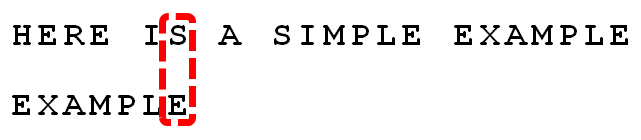2.依然从尾部开始比较，发现 'P' 与 'E' 不匹配，所以 'P' 是”坏字符”。但是， 'P' 包含在模式串 "EXAMPLE" 之中。因为 'P' 这个“坏字符”对应着模式串的第 6$6$$6$ 位（从 0$0$$0$ 开始编号），且在模式串中的最右出现位置为 4$4$$4$ ，所以，将模式串后移6 - 4 = 2位，两个 'P' 对齐。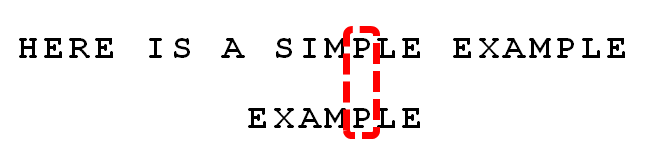3.依次比较，得到 “MPLE” 匹配，称为”好后缀”（ goodsuffix$goodsuffix$$good suffix$ ），即所有尾部匹配的字符串。注意， "MPLE" 、 "PLE" 、 "LE" 、 "E" 都是好后缀。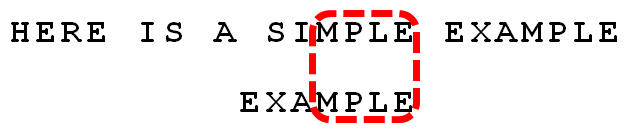4.发现 'I' 与 'A' 不匹配： 'I' 是坏字符。如果是根据坏字符规则，此时模式串应该后移2 - (-1) = 3位。问题是，有没有更优的移法？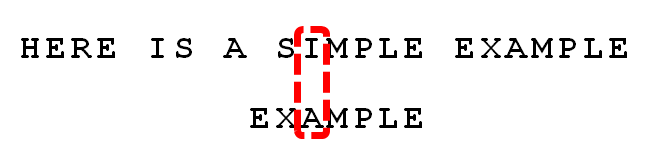5.更优的移法是利用好后缀规则：当字符失配时，后移位数 = 好后缀在模式串中的位置 - 好后缀在模式串中上一次出现的位置，且如果好后缀在模式串中没有再次出现，则为 −1$-1$$-1$ 。

所有的“好后缀”（ "MPLE" 、 "PLE" 、 "LE" 、 "E" ）之中，只有 'E' 在 “EXAMPLE” 的头部出现，所以后移6 - 0 = 6位。

可以看出，“坏字符规则”只能移 3$3$$3$ 位，“好后缀规则”可以移 6$6$$6$ 位。每次后移这两个规则之中的较大值。这两个规则的移动位数，只与模式串有关，与原文本串无关。6.继续从尾部开始比较， 'P' 与'E' 不匹配，因此 'P' 是“坏字符”，根据“坏字符规则”，后移 6 - 4 = 2位。因为是最后一位就失配，尚未获得好后缀。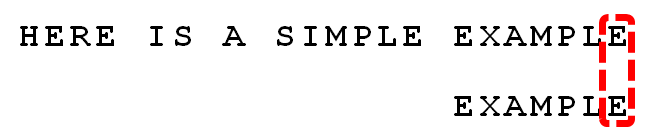由上可知， BM$BM$$BM$ 算法不仅效率高，而且构思巧妙，容易理解。

5. 扩展2：Sunday算法

上文中，我们已经介绍了 KMP$KMP$$KMP$ 算法和 BM$BM$$BM$ 算法，这两个算法在最坏情况下均具有线性的查找时间。但实际上， KMP$KMP$$KMP$ 算法并不比最简单的 C$C$$C$ 库函数 strstr() 快多少，而 BM$BM$$BM$ 算法虽然通常比 KMP$KMP$$KMP$ 算法快，但 BM$BM$`